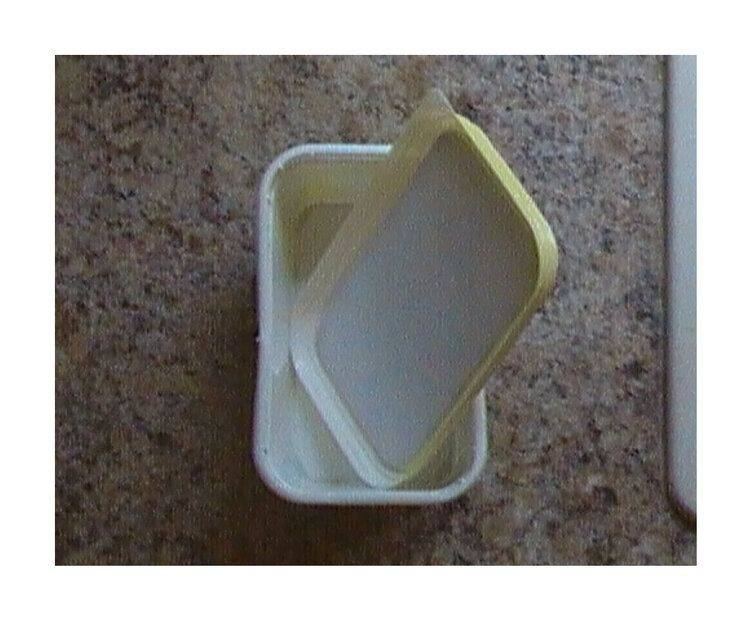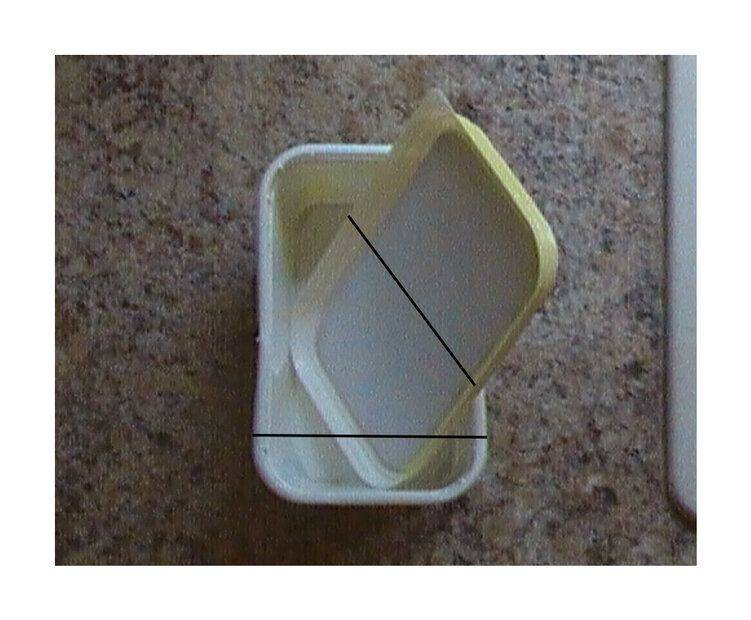# Compensating for a bias - a simple geometry/algebra problem:

• B
Gold Member
TL;DR Summary
Compensating for bias - a simple geometry/algebra problem:
There is this slight bias, that I will explain in a moment. But it is quite easy to do a calculation to compensate for this bias and show that it makes next to no difference. Could I ask people to check that the explanation and calculation are correct please?

The bias, and the calculation to correct for the bias

If you view a rectangle at an angle it makes it look less wide and less long. Let's call what the width and length of the rectangle appear to be, when viewed at an angle, the "apparent width" and the "apparent length", respectively.

In order to study what the rectangle's width and length are reduced down to, by viewing it at an angle, it is an idea to also have a copy of this rectangle which you don't view at an angle. Let's call this the "reference rectangle". Let's call the rectangle that you are viewing at an angle the "angled rectangle".

I am interested in what the width has reduced down to, expressed as a percentage (we will abbreviate this as WRDP). That is, the WRDP is obtained by dividing the "apparent width" of the "angled rectangle" by the width of the "reference rectangle" and then multiplying by 100. (Similarly for the length)

There was this instance where I used a margarine lid as my "angled rectangle" and the margarine tub as the "reference rectangle" (see figure A).Figure AFigure B

However, a margarine tub is actually a few millimeters smaller than a margarine lid - because a margarine lid fits over the margarine tub. This introduces a slight bias. At this point I need to introduce some notation:

We denote the "apparent width" of the margarine lid in the photo by ##W_{lid}^{photo}## (see line drawn in in figure B).
We denote the width of the margarine tub in the photo by ##W_{tub}^{photo}## (see line drawn in in figure B).

Then,

$$\text{the (biased) WRDP} = \dfrac{W_{lid}^{photo}}{W_{tub}^{photo}} \times 100 \qquad (Equation \; 1).$$

You correct for the slight bias by upscaling ##W_{tub}^{photo}## by the appropriate amount and then by using the upscaled version of ##W_{tub}^{photo}## in Equation 1 in place of ##W_{tub}^{photo}##. The amount you upscale by is determined by taking an actual margarine lid and tub and finding out how much bigger the lid is than the tub. I introduce more notation:

We denote the width of an actual margarine lid by ##W_{lid}##.
We denote the width of an actual margarine tub by ##W_{tub}##.

Let's denote the upscaled version of ##W_{tub}^{photo}## by ##\uparrow \hspace{-0.39em} W_{tub}^{photo}##. You want ##\uparrow \hspace{-0.4em} W_{tub}^{photo}## to be equal to the width the margarine lid would have in the photo if you weren't viewing it at an angle. With this in mind, it should fairly obvious that ##\uparrow \hspace{-0.31em} W_{tub}^{photo}## satisfies:

$$\dfrac{\uparrow \hspace{-0.34em} W_{tub}^{photo}}{W_{tub}^{photo}} = \dfrac{W_{lid}}{W_{tub}} .$$

From which we obtain a formula for the upscaled version of ##W_{tub}^{photo}##:

$$\uparrow \hspace{-0.34em} W_{tub}^{photo} = \dfrac{W_{lid}}{W_{tub}} \times W_{tub}^{photo}$$

We can now obtain the formula for the "corrected WRDP" by dividing ##W_{lid}^{photo}## by the upscaled version of ##W_{tub}^{photo}##:

$$\text{the corrected WRDP} = \dfrac{W_{lid}^{photo}}{\dfrac{W_{lid}}{W_{tub}} \times W_{tub}^{photo}} \times 100 = \dfrac{W_{tub}}{W_{lid}} \times \dfrac{W_{lid}^{photo}}{W_{tub}^{photo}} \times 100 .$$

Given that the ratio ##\dfrac{W_{tub}}{W_{lid}}## is very close to 1, the "(biased) WRDP" will be hardly changed.

We plug in the numbers. From Figure B the "(biased) WRDP" is about ##90 \%##. The width of an actual margarine tub is 9.2cm and the width of an actual margarine lid is 9.5cm, and so,

$$\text{the corrected WRDP} = \frac{9.2}{9.5} \times 90 \% = 87 \%$$

and so it has hardly changed!

A similar calculation can be done with regard to the length, with the same result that the answer is hardly changed!

Last edited: